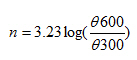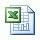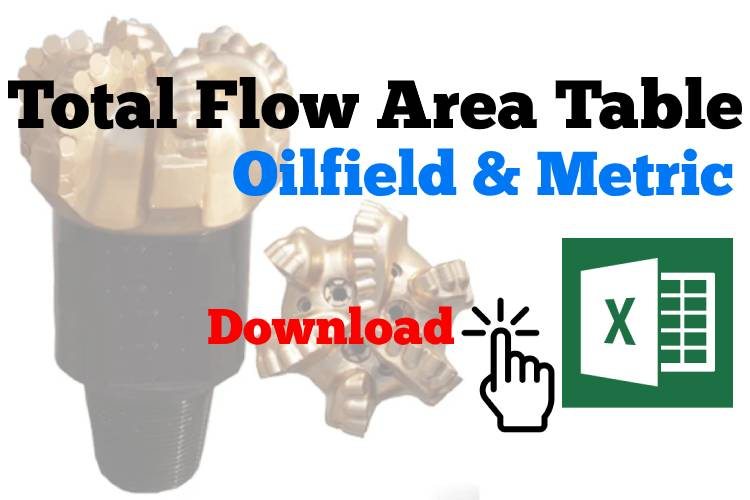## Surge and Swab Calculation Method 1

This is the first method to determine surge and swab pressure.

Surge is additional pressure due to pipe movement downward and swab is reduction of pressure due to upward movement of drill string.Bottom hole pressure is reduced due to swabbing effect.Bottom hole pressure is increased due to surging effect.

## Cutting Slip Velocity Calculation Method 2

This is another method to determine cutting slip velocity. The process of calculation is quite different from the first method however it is still straight forward calculation. It still gives you the following answers: annular velocity, cutting slip velocity and net velocity.

Let’s get started with this calculation method.

1. Determine nWhere;

n is the power law exponent.

Θ600 is a value at 600 viscometer dial reading.

Θ300 is a value at 300 viscometer dial reading.

## Cutting Slip Velocity Calculation Method 1

Cutting slip velocity is velocity of cutting that naturally falls down due to its density. In order to effectively clean the hole, effect of mud flow upward direction and mud properties must be greater than cutting slip velocity (settling tendency of cuttings). Otherwise, cutting will fall down and create cutting bed.

This calculation will show annular velocity, cutting slip velocity and net velocity so you can use as a reference for you hole cleaning indication.

There are 2 calculation methods and I will show the first method via this topic.

##Drilling Formula Calculation Sheet v1

When you one the file Drilling-formulas-Calculation-shee-V1.xls, you will see the fist page call index page which shows you all of formulas like this.

## Total Flow Area (TFA)Total Flow Area (TFA) is summation of nozzle areas which fluid can pass through a bit. When you consider about the TFA, you need to count all nozzles that you have in a bit or a reamer.Basically, you can determine flow area with a simple circle area formula.

Flow Area = (π × D2) ÷ 4

Where;

Area in square inch

π is a constant which approximately equates to 3.14159.

D is diameter in inch

Let’s make it easier for our life. Normally, a diameter of nozzle is reported in xx/32 inch. For example, a bit has 3 nozzles and each one of them has size of 20/32 inch.

The formula above can be simplified like this.

## Oilfield Unit

Flow Area = N2 ÷ 1303.8

Where;

Flow Area for each nozzle in square inch

N is nozzle size in number/32 inch.

In order to find the total flow area of each bit or reamer, you must add all area of each nozzle.

Example

The bit that has a total of 5 nozzles. Three nozzles have a diameter of 10/32 inch and other 2 nozzles are 12/32 inch diameter.

Determine the total flow area (TFA) of the bit.

By the definition, you must sum every nozzle together in order to get the TFA; therefore, you can apply the formula above into this form.

Total Flow Area = (102 + 102 + 102 + 122 + 122 )÷ 1303.8

Total flow area = 0.451 square inch

## Metric Unit

Flow Area = N2 × π ÷  4

Where;

Flow Area for each nozzle in square millimeter

N is nozzle size in millimeter .

Example

The bit that has a total of 5 nozzles. Three nozzles have a diameter of 7.94 mm and other 2 nozzles are 9.53 mm diameter.

Determine the total flow area (TFA) of the bit.

Total Flow Area = (7.942 + 7.942 + 7.942 + 9.532 + 9.53 2 ) × π ÷  4

Total flow area =  291 square mm